# Site map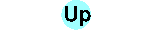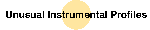## Instrumental profiles for different geometries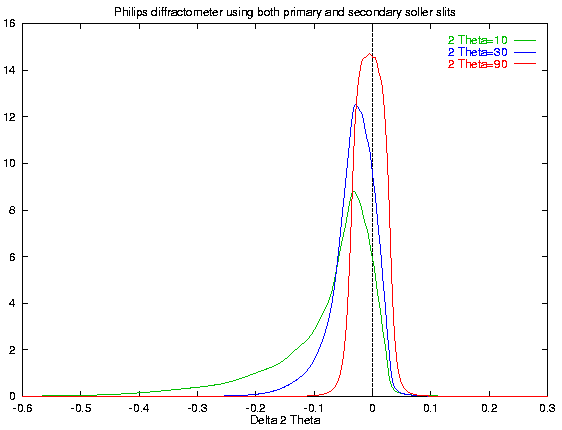explanation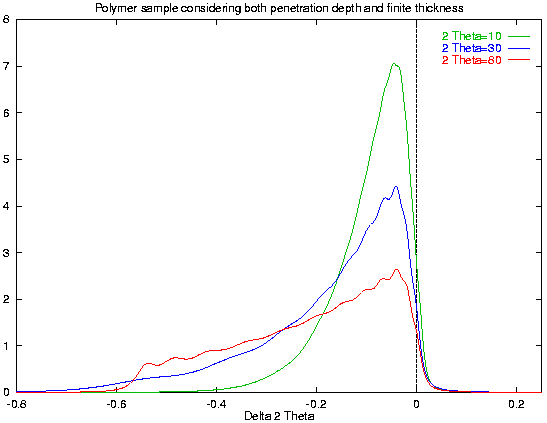explanation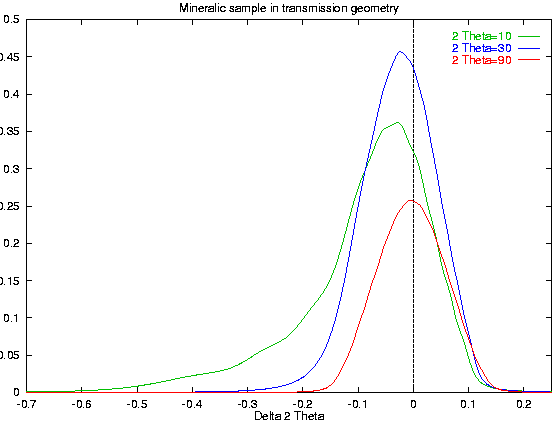explanation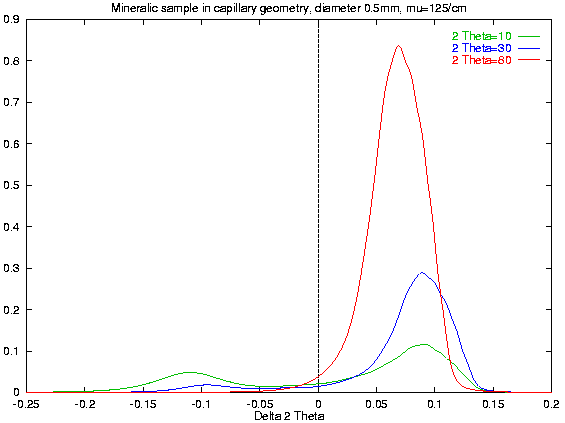explanation

#### 1st example

This example shows the common case of both primary and secondary soller slits collimator. It describes the slit system for the IUCR Rietveld Round Robin (PbSO4). We reached a Rwp value of 1 per cent better than the best result. The program was developed after the Rietveld Round Robin has finished. Therefore this result is not published. The command file for the computation is as follows:
```VERZERR=\val\kristall\ringtest\ringverz
R=173
rh=100
pi=2*acos(0)
% Feinfokusroehre
FocusH=8
FocusW=0.04
PColl=0.5*4.45*pi/180
SColl=0.5*4.45*pi/180
HSlitR=rh
HSlitW=(R-rh)*1*pi/180
SamplH=10
SamplW=20
DetW=0.2
DetH=15
MonR=R+51
zweiTheta=10
zweiTheta=14
zweiTheta=20
zweiTheta=28
zweiTheta=40
zweiTheta=65
zweiTheta=90
zweiTheta=120
zweiTheta=135
zweiTheta=150
zweiTheta=160
WMIN=10
WMAX=160
WSTEP=1
```

#### 2nd example

This is a measuring on a HZG 4 from the firm Seifert-FPM. Polymers commonly show reflections at very low angles. Therefore, primary and secondary soller slits are both in use. Please, remark the 1.6mm thick sample with a mu=3.3/cm! The detector is a semiconductor one for Kbeta separation. It's window (size of 8mm) was chosen instead of the monochromator crystal's hight and position. The command file is as follows:
```VERZERR=vjeh03
R=250
FocusH=10
FocusW=0.1
HSlitR=85
HSlitW=0.44
VSlitR=75
VSlitH=4
PColl=0.5/25
SamplD=12
DetW=0.22
DetH=8
MonR=R+8
zweiTheta=3
zweiTheta=4
zweiTheta=5
zweiTheta=6.5
zweiTheta=8
zweiTheta=10
zweiTheta=14
zweiTheta=20
zweiTheta=28
zweiTheta=40
zweiTheta=60
zweiTheta=80
T=1.6
D=T/0.53012
WMIN=3
WMAX=80
pi=2*acos(0)
WSTEP=2*sin(zweiTheta*pi/180)
GSUM=Y
```

#### 3th example

This was a measuring at an 3000TT diffractometer in transmission. The thickness of the specimen was estimated to 0.2mm. Because of the high linear attenuation coefficient of 250/cm the intensity is lowered for more than a magnitude. And there is a strong lowering of intensity for larger 2 theta angles. For compensation, this measuring was done without secondary soller slits. The command file is as follows:
```VERZERR=tran0205.ger
GEOMETRY=TRANSMISSION
R=250
D=0.08
T=0.2
HSlitR=R-150
HSlitW=0.2
VSlitR=R-80
VSlitH=17
FocusH=12
FocusW=0.04
DetH=14
MonR=R+50
DetW=0.5
SamplD=20
PColl=0.54/25
zweiTheta=6
zweiTheta=10
zweiTheta=16
zweiTheta=24
zweiTheta=34
zweiTheta=48
zweiTheta=60
zweiTheta=90
zweiTheta=120
WMIN=6
WMAX=120
pi=2*acos(0)
WSTEP=3*sin(pi*zweiTheta/180)
```

#### 4th example

This example shows the possibility of computation of capillary (or wire) geometry. The parameters capillary diameter and linear attenuation coefficient represent a realistic case for mineralic samples. Until now, we have not really used this capillary geometry. Please remark the double-peak at low angles, well known in Debye-Scherrer-films from high absorbing specimens. You also can see the common fact of increasing intensity with 2 theta angle. The command file is as follows:
```VERZERR=capillar
GEQ=capillar
GEOMETRY=CAPILLARY
R=250
FocusH=10
FocusW=0.1
HSlitR=85
HSlitW=0.44
VSlitR=75
VSlitH=4
PColl=0.5/25
SColl=0.5/25
DetW=0.22
DetH=8
MonR=R+8
zweiTheta=3
zweiTheta=4
zweiTheta=5
zweiTheta=6.5
zweiTheta=8
zweiTheta=10
zweiTheta=14
zweiTheta=20
zweiTheta=28
zweiTheta=40
zweiTheta=60
zweiTheta=80
WMIN=3
WMAX=80
pi=2*acos(0)
WSTEP=5*sin(zweiTheta*pi/180)
D=0.08
T=0.5
```